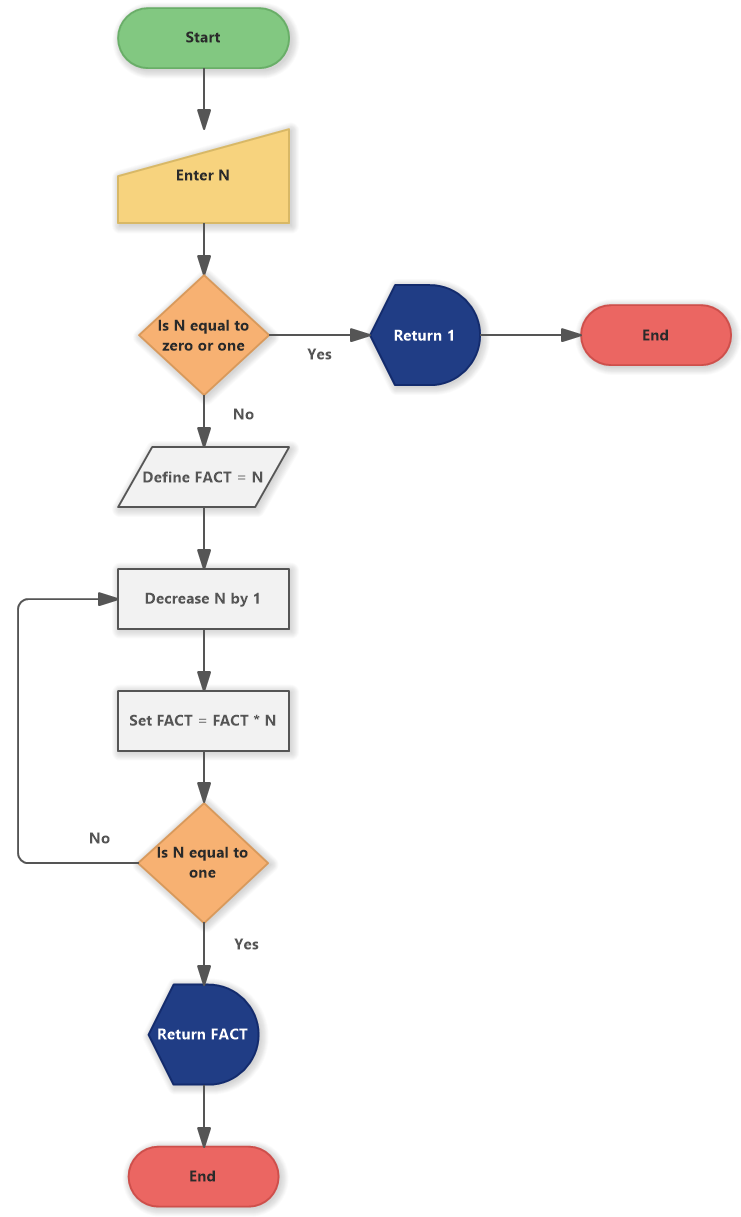# Factorial of a Number (Flowchart)

A flowchart example depicts an algorithm for factorial of a number. The program takes an input of number N from a user and calculates the factorial of it.

## Flowchart for Factorial of a Number

This flowchart example describes a step-by-step algorithm on how to calculate factorial of a given number.Factorial of a Number (Flowchart)

The flowchart for factorial of a number consists of the following steps:

• Start (Start End)
• Enter N (User Input)
• Is N equal to zero or one (Decision)
• Return 1 (Display)
• End (Start End)
• Decrease N by 1 (Process)
• Set FACT = FACT * N (Process)
• Define FACT = N (Data)
• Is N equal to one (Decision)
• Return FACT (Display)
• End (Start End)